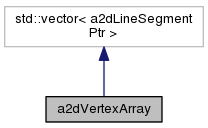wxArt2D
a2dVertexArray Class Reference

vertex array of line and arc segments. More...

`#include <polyver.h>`

Inheritance diagram for a2dVertexArray:[legend]
Collaboration diagram for a2dVertexArray:[legend]

## Public Member Functions

a2dVertexArray ()
constructor

a2dVertexArray (const a2dVertexArray &other)
constructor

a2dVertexArray (const a2dVertexList &other)

~a2dVertexArray ()
destructor

a2dVertexArrayoperator= (const a2dVertexArray &other)
operator =

a2dVertexArrayoperator= (const a2dVertexList &other)

a2dLineSegmentPtr GetPreviousAround (wxUint32 index) const
get the previous segment as a polygon ( GetLast() is no previous )

a2dLineSegmentPtr GetNextAround (wxUint32 index) const
get the next segment as a polygon ( GetFirst() is no next )

a2dLineSegmentPtr Item (wxUint32 index)

a2dLineSegmentPtr Item (wxUint32 index) const

void RemoveAt (size_t index)

void Insert (a2dLineSegment *segment, size_t index)

double Length () const
calculate length of path

void AddPoint (const a2dPoint2D &point, bool atEnd=true)
add point to end or begin

void AddPoint (double x, double y, bool atEnd=true)
add point to end or begin

void SetPointAdjustArcs (unsigned int n, double x, double y, bool polygon)
sets a point of a segment and adjusts arc it midpoints.

void SetPointAdjustArcs (a2dLineSegmentPtr seg, double x, double y, bool polygon)
sets a point of a segment and adjusts arc it midpoints.

double CalcArea () const

void ConvertToLines (double aberation=0)
Convert complex segments to line segments.

void Transform (const a2dAffineMatrix &world)
transform all segments with given matrix More...

bool HasArcs () const
return true if there are a2dArcSegment segments.

a2dBoundingBox GetBbox (const a2dAffineMatrix &lworld=a2dIDENTITY_MATRIX)
return a boundingbox of a transformed vertexarray

a2dVertexArrayContour (double distance, a2dPATH_END_TYPE pathtype)
create a contour around polygon/polyline

a2dVertexArrayConvertSplinedPolygon (double Aber) const
Spline conversion for polygon.

a2dVertexArrayConvertSplinedPolyline (double Aber) const
Spline conversion for polyline.

a2dVpathConvertToVpath (bool arc, bool closed=false)
return converted vector Vpath, arc segments stay intact if arc is true

a2dHit HitTestPolygon (const a2dPoint2D &ptest, double margin)
extensive hittesting on vertex list seen as polygon. More...

a2dHit HitTestPolyline (const a2dPoint2D &ptest, double margin)
extensive hittesting on vertex list seen as polyline. More...

bool RemoveRedundant (bool polygon)
line segments ( not arcs ) with same point are removed

a2dVertexListGetRedundant (bool polygon, double smallest=0)
line segments ( not arcs ) with same point are returned

## Detailed Description

vertex array of line and arc segments.

Holds a wxArray of a2dLineSegment objects. Drawing the sequence of segments, represents the form of the shape. Shape depends on the type of segment and style of that segment. There are two type, straight line segments and arc segments

Definition at line 494 of file polyver.h.

## Member Function Documentation

 double a2dVertexArray::CalcArea ( ) const

calculate the area of simple polygons (not selfintersecting) coordinates may be negatif

Definition at line 781 of file polyver.cpp.

 a2dHit a2dVertexArray::HitTestPolygon ( const a2dPoint2D & ptest, double margin )

extensive hittesting on vertex list seen as polygon.

Parameters
 ptest point to test against polygon. margin point with this margin around.
```This function checks if a point is inside, outside or on the stroke of a polygon.
```

This is done intersecting a vertical line through the test point with every edge of the polygon. Then the number of intersections above (y+) of the test point is counted +1 for left to right and -1 for right to left lines. There is an almost identical function for point list polygons. These functions should be kept identical except of the first two lines the handle lists or arrays.

Definition at line 1254 of file polyver.cpp.

 a2dHit a2dVertexArray::HitTestPolyline ( const a2dPoint2D & ptest, double margin )

extensive hittesting on vertex list seen as polyline.

Parameters
 ptest point to test against polyline. margin point with this margin around.

Definition at line 1453 of file polyver.cpp.

 void a2dVertexArray::Transform ( const a2dAffineMatrix & world )

transform all segments with given matrix

Remarks
complex segments will be broken down to lines.

Definition at line 823 of file polyver.cpp.

The documentation for this class was generated from the following files:
a2dVertexArray Class Reference -- Sun Oct 12 2014 17:04:51 -- Sun Oct 12 2014 -- 1.8.5 -- wxArt2D -- . -- Main Page Reference Documentation